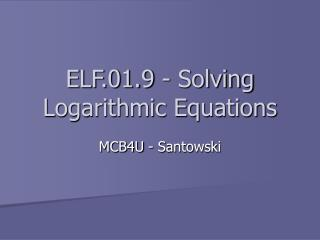DownloadDownload PresentationELF.01.9 - Solving Logarithmic Equations

# ELF.01.9 - Solving Logarithmic Equations

Télécharger la présentation## ELF.01.9 - Solving Logarithmic Equations

- - - - - - - - - - - - - - - - - - - - - - - - - - - E N D - - - - - - - - - - - - - - - - - - - - - - - - - - -
##### Presentation Transcript

1. ELF.01.9 - Solving Logarithmic Equations MCB4U - Santowski

2. (A) Introduction to Logarithmic Equations • Many measurement scales used for naturally occurring events like earthquakes, sound intensity, and acidity make use of logarithms • Additionally, since we know how logarithms and exponents are related, many applications involving exponents can also be mathematically analyzed using logarithms • So in working with these types of problems, we need to know how to solve logarithmic equations

3. (B) Example • The magnitude, R, of an earthquake on the Richter scale is given by the equation R = log(a/T) + B, where a is the amplitude of the vertical ground motion (measured in microns), T is the period of the seismic wave (measured in seconds) and B is a factor that accounts for the weakening of the seismic waves. Find the amplitude of the vertical ground motion for an earthquake that measured 6.3 on the Richter scale, and the period of the seismic wave was 1.6 seconds and B = 4.2

4. (B) Example • Givens: • R = log(a/T) + B • R = 6.3 • T = 1.6 sec • B = 4.2 • 6.3 = log(a/1.6) + 4.2 • 2.1 = log(a/1.6) •  now recall converting logs to exponents • 10(2.1) = a/1.6 • 125.89 = a/1.6 • 201.4 microns = a • Therefore, the amplitude of the ground wave would be approx 201 microns

5. (C) Solving Simple Log Equations • Solve the following equations:

6. (D) Internet Links to Simple Equations • Now work through the following worksheet to reinforce your skills with simple logarithms: • From edHelper.com - Logarithms

7. (E) Strategies for Solving Harder Logarithmic Equations • the two key ideas in solving logarithmic equations are: • (1) get both sides of the equation to be a single logarithmic expression • (2) both sides must have logarithms • the key tools you will use to work through logarithmic equations are the three laws - product law, quotient law and power law.

8. (E) Examples • Solve and verify and state the restrictions for log3(x) - log3(4) = log3(12) • Solve and verify and state the restrictions for log6(x + 3) + log6(x - 2) = 1 • Solve and verify and state the restrictions for log2(3x + 2) = 3 - log2(x - 1)

9. We will work out the following examples on a GDC: Graph y1 = log(x) – log(4) Graph y2 = log(12) Find their intersection Which occurs when x = 48 (F) Connections to Graphs

10. But what happens when the base of the logarithm is different??? The GDC works in base 10!!!! We will use a different base (e)  ln (loge) on a GDC: Graph y1 = ln(x) – ln(4) Graph y2 = ln(12) Find their intersection …. Which occurs when x = 48 (although the y-value has changed)  so base does NOT matter for the solution of x (F) Connections to Graphs

11. Recall that solving equations also relates to finding x-intercepts  how does that apply here? If we rearrange the equation, we get  log(x) – log(4) – log(12) = 0 Now graph this rearranged equation and find the x-intercept(s) (F) Connections to Graphs

12. (G) Internet Links • Solving Logarithmic Equations Lesson - From PurpleMath • SOLVING LOGARITHMIC EQUATIONS - from SOSMath • College Algebra Tutorial on Logarithmic Equations from WTAMU

13. (D) Homework • Nelson text, page 146, Q3,6,7,8,12,16-19